Over a million developers have joined DZone.{{announcement.body}}
{{announcement.title}}

DZone's Guide to

# Standardization in Lasso

We venture into the wild west of data and learn how to wrangle and standardize data with a lasso regression. Read on to learn how to visualize this data as well!

· Big Data Zone ·
Free Resource

Comment (0)

Save
{{ articles.views | formatCount}} Views

Cloudera Data Flow, the answer to all your real-time streaming data problems. Manage your data from edge to enterprise with a no-code approach to developing sophisticated streaming applications easily. Learn more today.

The lasso regression is based on the idea of solving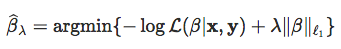where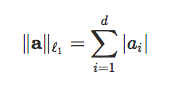for any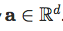In a recent post, we've seen computational aspects of the optimization problem. But I went quickly through the story of the l1-norm. Because it means, somehow, that the value of ß1 and ß2 should be comparable. Somehow, with two significant variables, with very different scales, we should expect orders (or relative magnitudes) of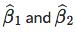to be very very different. So people say that it is, therefore, necessary to center and reduce (or standardize) the variables.

Consider the following (simulated) dataset

``````Sigma = matrix(c(1,.8,.2,.8,1,.4,.2,.4,1),3,3)
n = 1000
library(mnormt)
X = rmnorm(n,rep(0,3),Sigma)
set.seed(123)
df = data.frame(X1=X[,1],X2=X[,2],X3=X[,3],X4=rnorm(n),
X5=runif(n),X6=exp(X[,3]),
X7=sample(c("A","B"),size=n,replace=TRUE,prob=c(.5,.5)),
X8=sample(c("C","D"),size=n,replace=TRUE,prob=c(.5,.5)))
df\$Y = 1+df\$X1-df\$X4+5*(df\$X7=="A")+rnorm(n)
X = model.matrix(lm(Y~.,data=df))``````

Use the following colors for the graphs and the value of lambda.

``````library("RColorBrewer")
colrs = c(brewer.pal(8,"Set1"))[c(1,4,5,2,6,3,7,8)]
vlambda=exp(seq(-8,1,length=201))``````

The first regression we can run is a non-standardized one

``````library(glmnet)
lasso = glmnet(x=X,y=df[,"Y"],family="gaussian",alpha=1,lambda=vlambda,standardize=FALSE)``````

We can visualize the graphs of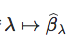using the following code:

``````idx = which(apply(lasso\$beta,1,function(x) sum(x==0))&lt;200)
plot(lasso,col=colrs,'lambda',xlim=c(-5.5,2.3),lwd=2)
legend(1.2,.9,legend=paste('X',0:8,sep='')[idx],col=colrs,lty=1,lwd=2)``````At least, observe that the most significant variables are the ones that were used to generate the data.

Now, consider the case that we standardize the data

``lasso = glmnet(x=X,y=df[,"Y"],family="gaussian",alpha=1,lambda=vlambda,standardize=TRUE)``

The graphs ofThe graph is (strangely) very similar to the previous one. Except perhaps for the green curve. Maybe that categorical is not similar to the continuous variables... because, somehow, standardization of categorical variables might be not natural...

Why not consider some home-made function? Let us transform (linearly) all variable in the X matrix (except the first one, which is the intercept):

``````Xc = X
for(j in 2:ncol(X)) Xc[,j]=(Xc[,j]-mean(Xc[,j]))/sd(Xc[,j])``````

Now, we can run our lasso regression on that one (with the intercept since all the variables are centered, but y)

``lasso = glmnet(x=Xc,y=df\$Y,family="gaussian",alpha=1,intercept=TRUE,lambda=vlambda)``

The plot is now:

``````plot(lasso,col=colrs,"lambda",xlim=c(-6.7,1.3),lwd=2)
idx = which(apply(lasso\$beta,1,function(x) sum(x==0))&lt;length(vlambda))
legend(.15,.45,legend=paste('X',0:8,sep='')[idx],col=colrs,lty=1,bty=&quot;n&quot;,lwd=2)``````Actually, why not also center the y variable, and remove also the intercept:

``````Yc = (df[,"Y"]-mean(df[,"Y"]))/sd(df[,"Y"])
lasso = glmnet(x=Xc,y=Yc,family="gaussian",alpha=1,intercept=FALSE,lambda=vlambda)``````Hopefully, those graphs are very consistent (and if we use those for variable selection, they suggest using variables that were actually used to generate the dataset). And having qualitative and quantitative variables is not a big deal. But still, I do not feel comfortable with the differences...

Cloudera Enterprise Data Hub. One platform, many applications. Start today.

Topics:
big data ,lasso regression ,data standardization ,data visualization

Comment (0)

Save
{{ articles.views | formatCount}} Views

Published at DZone with permission of

Opinions expressed by DZone contributors are their own.

# {{ parent.title || parent.header.title}}

{{ parent.tldr }}

{{ parent.urlSource.name }}